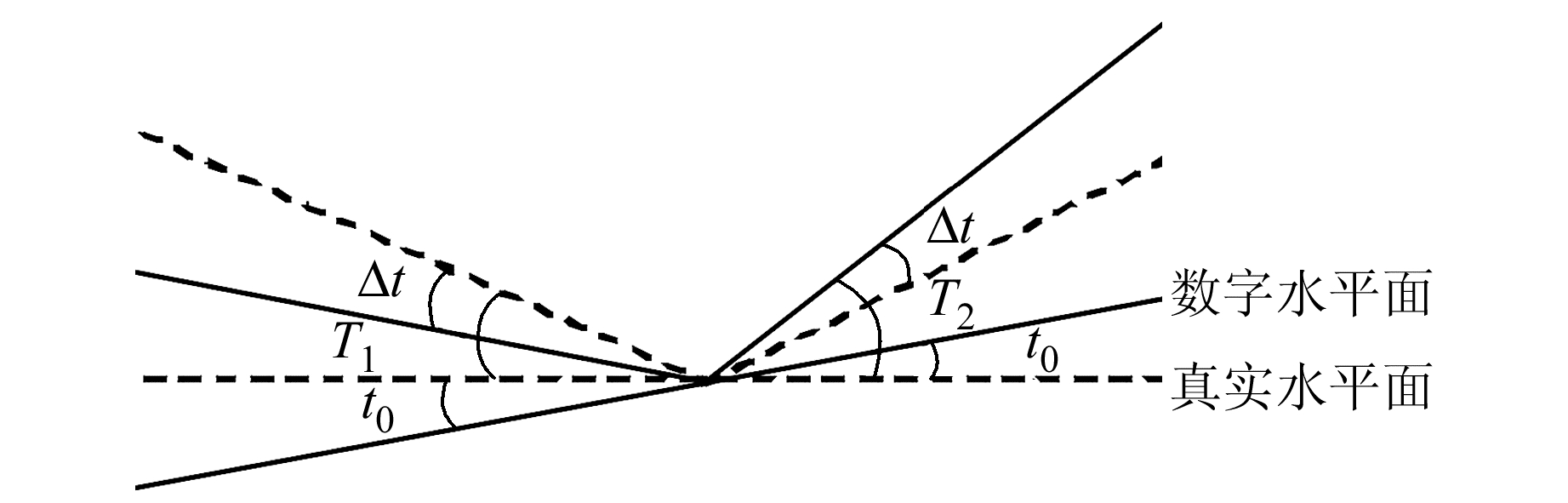﻿ CG-5重力仪倾斜传感器检验调整原理解析及应用文章快速检索 高级检索
 大地测量与地球动力学2019, Vol. 39Issue (4): 432-436  DOI: 10.14075/j.jgg.2019.04.019### 引用本文CAO Jinguo, LI Yongming, GAO Xu'na, et al. Adjusting Principle of CG-5 Gravimeter Tilt Sensor Parameters: Analysis and Applications[J]. Journal of Geodesy and Geodynamics, 2019, 39(4): 432-436.### 第一作者简介

CAO Jinguo, senior engineer, majors in gravity measurements and data processing, E-mail: jing12345678525@sina.com.

### 文章历史

CG-5重力仪倾斜传感器检验调整原理解析及应用

1. 中国人民解放军96633部队，北京市，100096;
2. 中国人民解放军96711部队，安徽省池州市，247100

CG-5重力仪是加拿大Scintrex公司制造的数字重力仪，其精度可靠、性能稳定、操作简便，广泛用于矿藏勘探、地质填图、地震预报及区域重力研究等。仪器使用过程中需要定期对倾斜传感器零位补偿和灵敏度进行检验并视情况调整，使倾斜传感器精确反映仪器的真实倾斜状况。解析重力仪倾斜传感器检验调整原理，对于掌握仪器性能、改进检验调整方法、解决仪器使用中的问题乃至仪器二次开发利用等都至关重要。目前国内尚无关于该仪器检验调整原理方面的文献资料，本文从仪器倾斜角度与重力读数的关系入手，推导解析仪器检验调整的原理。

1 倾斜传感器检验调整步骤

1.1 零位补偿检验调整步骤

1.2 灵敏度检验调整步骤

2 倾斜传感器检验调整原理解析

2.1 倾斜传感器零位补偿检验调整原理解析

 ${T_1} = \left[ {\left( {{S_1} - {t_0}} \right) \times 0.000\;076\;295 - 2.5} \right] \times L$ (1)
 ${T_2} = \left[ {\left( {{S_2} - {t_0}} \right) \times 0.000\;076\;295 - 2.5} \right] \times L$ (2)图 1 倾斜传感器零位补偿检验调整关系 Fig. 1 The tilt sensor offset adjusting interrelation

2种倾斜状态下，敏感的仪器重力传感器将电压信息转换为重力信息，进行温度补偿和固体潮改正后的相对重力读数分别为g1g2。同一位置2个相对重力读数产生差异的原因是仪器倾斜造成的，即

 ${g_1} - {g_2} = \left( {g - g_\Delta ^1} \right) - \left( {g - g_\Delta ^2} \right)$ (3)

 ${g_\Delta } = \gamma \times \left( {1 - \cos \left( {T + \Delta t} \right)} \right)$ (4)

 $\cos \left( {{T_2} + \Delta t} \right) - \cos \left( {{T_1} + \Delta t} \right) = \frac{{{g_1} - {g_2}}}{\gamma }$ (5)

 $A\cos \Delta t + B\sin \Delta t = \frac{{{g_1} - {g_2}}}{\gamma }$ (6)

 $\begin{array}{*{20}{c}} {A = \cos {T_2} - \cos {T_1}}\\ {B = \sin {T_1} - \sin {T_2}} \end{array}$

 $\sqrt {{A^2} + {B^2}} \sin \left( {\Delta t + \varphi } \right) = \frac{{{g_1} - {g_2}}}{\gamma }$ (7)

 $\varphi = \arcsin \left( {\frac{B}{{\sqrt {{A^2} + {B^2}} }}} \right)$

 $\Delta t = \arcsin \left( {\frac{{\left. {{g_1} - {g_2}} \right)}}{{\gamma \sqrt {{A^2} + {B^2}} }}} \right) - \varphi$ (8)

 $t = {t_0} + \Delta t$ (9)

2.2 倾斜传感器灵敏度检验调整原理解析

 $\begin{array}{*{20}{c}} {\cos \left[ {{k_1}\left( {{L_0} + \Delta L} \right)} \right] - }\\ {\cos \left[ {{k_2}\left( {{L_0} + \Delta L} \right)} \right] = \frac{{{g_2} - {g_1}}}{\gamma }} \end{array}$ (10)

 ${k_1} = \left[ {\left( {{S_1} - t} \right) \times 0.000\;076\;295 - 2.5} \right]$ (11)
 ${k_2} = \left[ {\left( {{S_2} - t} \right) \times 0.000\;076\;295 - 2.5} \right]$ (12)

 $L'' = {L_0} + \Delta L = 206\;265'' \times \sqrt {\frac{{2\left( {{g_2} - {g_2}} \right)}}{{\gamma \left( {k_1^2 - k_2^2} \right)}}}$ (13)

3 倾斜传感器检验调整过程解析 3.1 倾斜传感器零位补偿检验调整流程解析

3.2 倾斜传感器灵敏度检验调整流程解析

 $t = \frac{{t''}}{{{L_0} \times 0.000\;076\;295}}$ (14)

4 倾斜传感器检验调整解析参数结果比对表 1 X方向倾斜传感器零位补偿值比对结果 Tab. 1 The comparison of X-axis tilt sensor offset between instrument internal calculation and analysis calculation表 2 Y方向倾斜传感器零位补偿值比对结果 Tab. 2 The comparison of Y-axis tilt sensor offset between instrument internal calculation and analysis calculation表 3 X方向倾斜传感器灵敏度值比对 Tab. 3 The comparison of X-axis tilt sensor sensitivity between instrument internal calculation and analysis calculation表 4 Y方向倾斜传感器灵敏度值比对 Tab. 4 The comparison of Y-axis tilt sensor sensitivity between instrument internal calculation and analysis calculation

5 倾斜传感器检验调整限差设置

5.1 零位补偿偏差和灵敏度偏差对重力倾斜改正的影响 5.1.1 零位补偿偏差对重力倾斜改正的影响

 ${\rm{d}}\left( {{g_\Delta }} \right) = \frac{{980\;600\sin T \times {\rm{d}}\left( {\Delta t} \right)}}{{206\;265}}$ (15)表 5 不同倾斜状态下零位补偿偏差对重力倾斜改正的影响 Tab. 5 The influence of different axis tilt sensor offset deviation on gravity correction for inclination
5.1.2 灵敏度偏差对重力倾斜改正的影响

 $T = \left[ {\left( {S - \frac{t}{{CL}}} \right) \times C - 2.5} \right] \times L$ (16)

 ${\rm{d}}\left( {{g_\Delta }} \right) = \frac{{980\;600 \times \left( {SC - 2.5} \right) \times \sin T \times {\rm{d}}\left( {\Delta L} \right)}}{{206\;265}}$ (17)表 6 不同倾斜状态下灵敏度偏差对重力倾斜改正的影响 Tab. 6 The influence of different axis tilt sensor sensitivity deviation on gravity correction for inclination
5.2 零位补偿和灵敏度限差设置要求

6 结语

  SCINTREX Limited. CG-5 Scintrex Autograv System Operation Manual[Z]. USA, 2007 (0)  曹金国, 王建斌, 戴山岭. 重力场扰动位泰勒展开式低阶项应用研究[J]. 测绘通报, 2014(1): 54-56 (Cao Jinguo, Wang Jianbin, Dai Shanling. Research on the Application of Low-Order Taylor Expansion for Gravity Field Disturbing Potential[J]. Bulletin of Surveying and Mapping, 2014(1): 54-56) (0)  曹金国, 李永明, 王存军, 等. CG-5重力仪传感器信号应用解析[J]. 北京测绘, 2018, 32(1): 62-65 (Cao Jinguo, Li Yongming, Wang Cunjun, et al. The Application Analysis of CG-5 Gravimeter Sensor Signal[J]. Beijing Surveying and Mapping, 2018, 32(1): 62-65) (0)  王庆宾, 吴晓平. 相对重力测量值的改正[J]. 测绘学院学报, 2001, 18(增1): 8-10 (Wang Qingbin, Wu Xiaoping. The Correction for Relatively Gravity Measurement[J]. Journal of Institute of Surveying, 2001, 18(S1): 8-10) (0)  徐菊生, 刘序俨. 重力测量学[M]. 北京: 地震出版社, 1993 (Xu Jusheng, Liu Xuyan. Gravimetry[M]. Beijing: Seismological Press, 1993) (0)
Adjusting Principle of CG-5 Gravimeter Tilt Sensor Parameters: Analysis and Applications
CAO Jinguo1LI Yongming1     GAO Xu'na1     QI Yufei1     CHE Shibao2
1. 96633 Troops of PLA, Beijing 100096, China;
2. 96711 Troops of PLA, Chizhou 247100, China
Abstract: The paper analyses the principle of tilt sensor's zero compensation and sensitivity adjustment according to adjusting procedures of the CG-5 gravimeter tilt sensor parameters. An experiment is performed to verify the principle. The results show that the principle analyzed in this paper is correct. Then, a basis for adjusting tolerance of the tilt sensor parameters is proposed. The principle analysis will be helpful for mastering the CG-5 gravimeter. Furthermore, it is useful for optimizing the instrument and secondary development.
Key words: CG-5 gravimeter; tilt sensor; adjusting; principle analysis